# Module 4: Drivers Of Reactions | Year 11 Chemistry Guide

Struggling with Year 11 Drivers of Reactions? In this Guide, we help you become proactive rather than reactive by guiding you through this subject.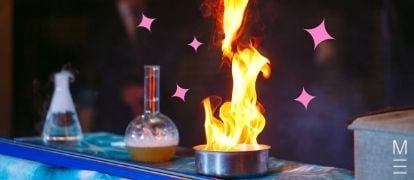So far, you’ve learnt a lot about different chemical reactions, but have not covered exactly why these reactions occur. In this article, we introduce the drivers of reactions – the reasons why a chemical reaction will take place.

This topic introduces new concepts that help explain whether or not certain reactions take place, including enthalpy, entropy and Gibb’s free energy. This topic also explores Thermodynamics, which deals with the laws that govern the movement of heat and energy.

## In this drivers of reactions guide, we discuss:A free pocket-sized cheatsheet, so you're always prepared.

## Energy Changes in Chemical Reactions

A chemical reaction always involves the absorption or release of energy. Using a few simple formulae, we can perform calculations that allow us to quantify how much energy is associated with a chemical reaction.

### Enthalpy Change and Calorimetry

When we measure the release or absorption of energy in a chemical reaction, what we are really measuring is the change in energy of the products compared to the reactants. There are two important quantities to consider, heat and enthalpy.

• Heat $$q$$ is the total energy absorbed or released as heat during the reaction. It is measured in joules ($$\text{J}$$).
• Enthalpy $$H$$ is one measure of how much energy a particular chemical species contains. It is measured in kilojoules per mole ($$\text{kJ mol}^{-1}$$).

During a chemical reaction, the reactants are converted into the products. The reactants and products will contain different amounts of energy, i.e. their enthalpy will be different. We cannot, however, measure the enthalpy of the reactants and products directly (the absolute enthalpy) and instead need to measure the change in enthalpy $$\Delta H$$. This difference in enthalpy is due to heat energy being absorbed or released to the surrounding environment.

We can classify reactions into two types:

• Exothermic reactions release heat and have a negative enthalpy change ($$\Delta H < 0$$). This means the products have a lower enthalpy than the reactants.
• Endothermic reactions absorb heat and have a positive enthalpy change ($$\Delta H > 0$$). This means the products have a higher enthalpy than the reactants.

We can describe the heat involved in a reaction, the temperature change and the change in enthalpy using the equations below:

$$q=mc \Delta T$$

$$q$$ is the heat energy produced or absorbed by a reaction, $$m$$ is the mass of a substance undergoing a temperature change, $$c$$ is the specific heat capacity of the substance, and $$\Delta T$$ is the change in temperature of the substance.

$$\Delta H= \frac{-q}{n}$$

$$\Delta H$$ represents the change in enthalpy of the reaction, $$q$$ is the heat energy produced or absorbed by a reaction in kilojoules ($$\text{kJ}$$), and $$n$$ is the number of moles of a substance involved in the chemical reaction.

Now that we know these essential formulae, we can have a look at calorimetry, which is an experimental technique that allows us to measure heat. There are two types of calorimetry you will need to be familiar with, solution calorimetry and combustion calorimetry.

### Combustion Calorimetry

A diagram showing the typical setup of a calorimeter used to investigate the enthalpy change of a combustion reaction is shown below: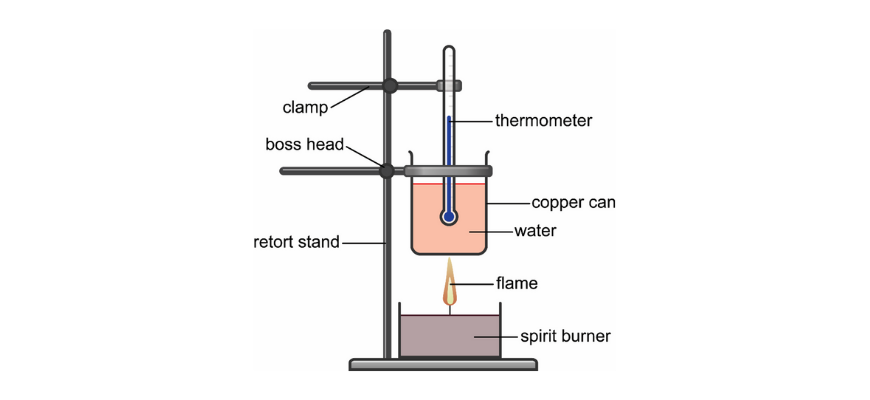A spirit burner containing a fuel (such as ethanol) is lit underneath a copper can containing a known mass of water. The energy produced by the reaction heats the water, and if we know the temperature change of the water, we can calculate the heat $$q$$ released by the combustion. If we know the amount of substance that combusted in moles, we can then work out the $$\Delta H$$ of the reaction.

### Solution Calorimetry

A similar process can be followed for solution calorimetry – a diagram showing the typical setup of a calorimeter used to investigate the enthalpy change of a reaction in solution is shown below.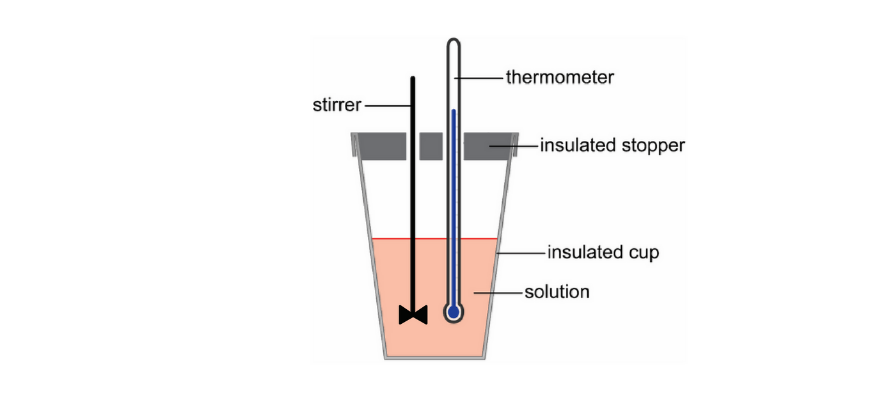A solution calorimeter will initially contain a known mass of water. A solute (such as $$NH_4Cl$$), is then dissolved in the water and the largest temperature change is recorded. The energy produced or absorbed during the dissolution changes the temperature of the water, and if we know the temperature change of the water and the amount of the substance added to it, we can calculate the heat and the $$\Delta H$$ of the process.

A solution calorimeter can also be used for measuring the enthalpy of neutralisation reactions, or other reactions that take place in solution. For example, you could begin with a solution of sodium hydroxide ($$NaOH_{(aq)}$$) and add a solution of hydrochloric acid ($$HCl_{(aq)}$$) to the calorimeter.

### Energy Profile Diagrams

When we talk about energy changes in chemical reactions, it can be difficult to visualise exactly what we are talking about. One way we can represent these energy changes is to use an energy profile diagram.

An energy profile diagram has two axes – energy on the $$y$$ axis and reaction coordinate on the $$x$$ axis. You can think of the “reaction coordinate” as the progress of the reaction.

In the case of an exothermic reaction, the energy of the products is less than the energy of the reactants, meaning the reaction releases heat as it proceeds. Since the total energy of the reaction has decreased, $$\Delta H$$ is negative. This information can be illustrated using an energy profile diagram as shown below: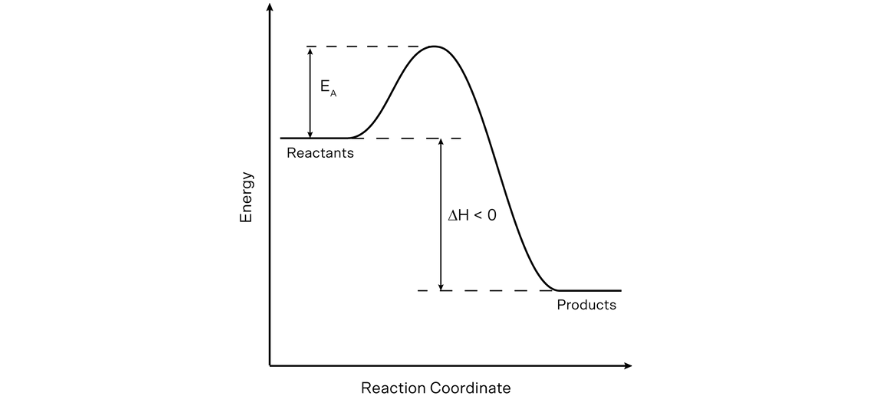The activation energy ($$E_A$$) of a chemical reaction can also be illustrated on an energy profile diagram, as can be seen in the diagram above. Remember, the activation energy is the amount of energy required for a chemical reaction to occur.

It is also important to consider what an energy profile diagram would look like for an endothermic reaction. Since heat is absorbed, the products of an endothermic reaction will have more energy than the reactants. As such, the total energy of the reaction has increased, and $$\Delta H$$ is positive. This is illustrated on the energy profile diagram below.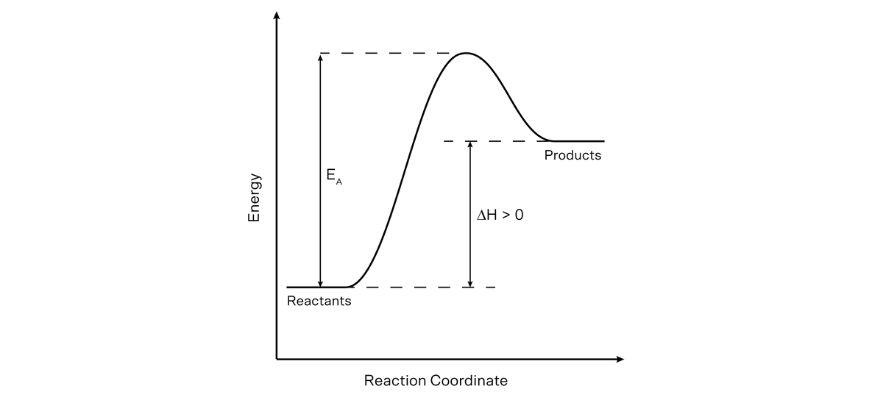## Catalyse a positive change in your Chemistry marks!

Our Year 11 Matrix Chemistry course will boost your marks on your terms. Find out how.

## Catalysts in Chemical Reactions

Catalysts play a vital role in many chemical processes, but what are they and how do they work? Let’s start with a definition.

A catalyst is a substance that increases the rate of a chemical reaction, and is not consumed in the process. This is because a catalyst provides an alternate reaction pathway with a lower $$E_A$$. The effect of a catalyst is illustrated on the energy profile diagram below: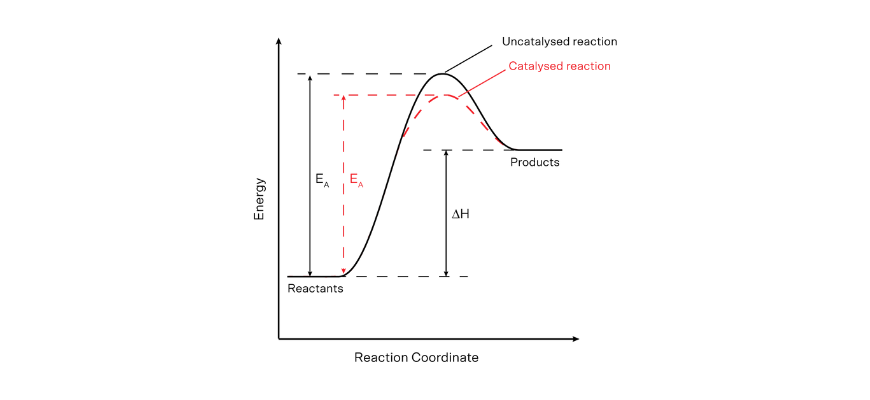A lower $$E_A$$ means that more collisions will have enough energy to exceed the activation energy barrier at a given temperature – this results in an increased rate of reaction.

An excellent analogy for a catalyst is the difference between climbing over a mountain and walking through the mountain using a tunnel. The tunnel is a lower energy pathway, and represents the catalysed reaction.

## Enthalpy and Hess’s Law

We have previously been talking about the enthalpy of reaction, $$\Delta H$$, which is the energy change that occurs due to a chemical reaction. Next, we’ll take a look at some different rules which apply to the enthalpy of a chemical reaction.

### The Law of Conservation of Energy

This law states that energy is conserved (stays the same). In other words, “energy can neither be created nor destroyed, it can only be transformed or transferred from one form to another”.

It is important to realise that chemical reactions do not violate this rule. For example, an exothermic reaction does not produce energy from nothing. Generally, you can think about the chemical energy being “stored” in chemical bonds. This is because different chemical bonds have a different amount of energy associated with them. For example, a $$C—H$$ bond has a bond enthalpy of $$413 \text{ kJ mol}^{–1}$$ and a $$C—O$$ bond has a bond enthalpy of $$358 \text{ kJ mol}^{–1}$$. If one is changed into the other during a chemical reaction, the difference in energy will be released.

Conversely, an endothermic chemical reaction does not generate higher energy products without an input of energy – the required energy is absorbed from the surroundings.

### Hess’s Law

Hess’s Law states that the total enthalpy change of a chemical reaction is the same, regardless of the path that is taken to convert reactants to products. Let’s explore this with the diagram below.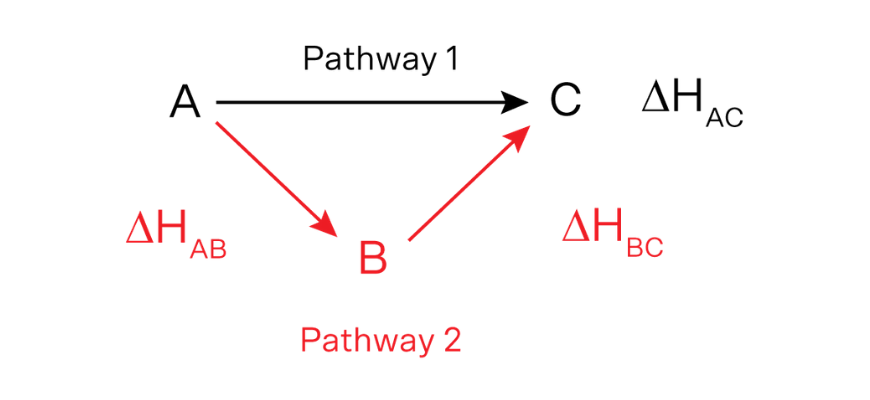In this diagram, the reactants ($$A$$) form products ($$C$$). Let’s say that this results in an enthalpy change of $$\Delta H_{AC}$$. Now consider that there is an alternative pathway (Pathway 2), where the reactants form an intermediate product ($$B$$) with an enthalpy change of $$\Delta H_{AB}$$. The intermediate product ($$B$$) is then converted to the final product ($$C$$) with an enthalpy change of $$\Delta H_{BC}$$

Hess’ Law tells us that the total change in enthalpy is the same for each pathway. That is $$\Delta H_{AB} + \Delta H_{BC} = \Delta H_{AC}$$

## Entropy and Gibbs Free Energy

So far we have covered the change in enthalpy – the energy change that occurs during a reaction. However, there are two other important factors that must be considered when deciding whether or not a reaction will occur spontaneously – the entropy change of the reaction and the Gibbs free energy change associated with the process.

### Entropy

Entropy is the term used to describe energy distribution within a system. If energy is more widely distributed, the entropy of the system increases. If energy is less widely distributed within a system, entropy decreases. Unlike enthalpy, we can measure the absolute entropy of any substance (Third Law of Thermodynamics).

Circumstances where entropy will increase are outlined below. For each circumstance, an example of a process where this occurs is listed. The opposite process in each example would decrease entropy.

• The number of particles within a system increases
• $$2O_{3(g)} ⟶ 3O_{2(g)}$$
• Particles within a system are mixed
• $$NaCl_{(s)} ⟶ NaCl_{(aq)}$$
• The volume of a gaseous system is increased
• A $$5$$ L container of gas expands to $$10$$ L
• A state change that results in more freedom of movement (entropy increases from solid to liquid/aqueous to gas)
• $$N_{2(l)} ⟶ N_{2(g)}$$
• The temperature of a system increases
• A container of gas is heated from $$25 °$$C to $$100 °$$C
• The complexity of the particles within the system increases
• 1 mole of $$C_6H_5CHCHCHO_{(l)}$$ will have a greater entropy than $$1$$ mole of $$Br_{2(l)}$$ at the same temperature

There are a lot of different circumstances here! You must be able to model each of these circumstances to explain why entropy increases in each case.

Importantly, the Second Law of Thermodynamics states that the entropy of the universe is always increasing. This means any localised decrease in entropy must be offset by an entropy increase elsewhere.

For example, if your freezer solidifies water to make ice, then the entropy of the water has decreased. This decrease is offset by a number of other processes that result in an increase in entropy, such as the heat produced at the back of your fridge warming the surroundings.

A consequence of the Second Law of Thermodynamics is that a reaction will be more likely to occur spontaneously if there is an increase in entropy.

### Entropy Changes in Reactions

Just as enthalpy changes during a chemical reaction, so too does entropy. We can calculate the standard entropy change of a reaction ($$\Delta S°_{rxn}$$) using the standard entropies values of the reactants and products ($$S°$$). To do this, you need to use the formula:

$$∆S°_{rxn}=∑S°_{products} – ∑S°_{reactants}$$

To use this formula, we sum the standard entropies of each of the products ($$∑S°_{products}$$)  and subtract the sum of the standard entropies of each of the reactants ($$∑S°_{reactants}$$).

### Reaction Spontaneity and Gibbs Free Energy

A spontaneous reaction is one that is capable of proceeding under a specific set of conditions without the continuous input of energy from an outside source.

We can determine if a reaction is spontaneous by considering the change in Gibbs Free Energy, $$\Delta G$$, using the equation below:

$$∆G°_{rxn}=∆H°_{rxn}- T∆S°_{rxn}$$

$$\Delta G =$$ change in Gibbs free energy in $$\text{kJ mol}^{-1}$$, $$\Delta H =$$ change in enthalpy in $$\text{kJ mol}^{-1}$$, $$\Delta S =$$ change in entropy in $$\text{kJ}\text{ mol}^{-1}\text{ K}^{-1}$$, and $$T =$$ temperature in $$K$$.

For all reactions:

• If $$\Delta G < 0$$, the reaction is spontaneous
• If $$\Delta G > 0$$, the reaction is not spontaneous
• If $$\Delta G = 0$$, then the reaction is at equilibrium

Given the necessary thermodynamic data, you can predict if a reaction will occur spontaneously at a given temperature by determining the change in Gibbs free energy. To use the Gibbs free energy equation, you must always convert temperature to kelvin and ensure that the entropy change is in kilojoules.

You should note that the term for entropy in this equation is temperature-dependant, so at higher temperatures, the contribution from the entropy term will have a greater influence on the Gibbs free energy value. The following table can be used to consider the effect of entropy, enthalpy and temperature on the change in Gibbs free energy (and hence reaction spontaneity).

 $$∆H < 0$$ $$∆H > 0$$ $$∆S > 0$$ Spontaneous at all temperatures $$∆G < 0$$ Spontaneous at high temperatures ($$T∆S$$is large) $$∆S < 0$$ Spontaneous at low temperatures ($$T∆S$$ is small) Non-spontaneous at all temperatures $$∆G > 0$$

© Matrix Education and www.matrix.edu.au, 2022. Unauthorised use and/or duplication of this material without express and written permission from this site’s author and/or owner is strictly prohibited. Excerpts and links may be used, provided that full and clear credit is given to Matrix Education and www.matrix.edu.au with appropriate and specific direction to the original content.